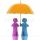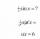# Triangle SAS

Calculate the area and perimeter of the triangle, if the two sides are 51 cm and 110 cm long and angle them clamped is 130 °.

Result

perimeter:  309.03 cm
triangle area:  2148.75 cm2

#### Solution:

Try calculation via our triangle calculator.Leave us a comment of example and its solution (i.e. if it is still somewhat unclear...):

Showing 0 comments:Be the first to comment!#### To solve this verbal math problem are needed these knowledge from mathematics:

Pythagorean theorem is the base for the right triangle calculator. Cosine rule uses trigonometric SAS triangle calculator. See also our trigonometric triangle calculator.

## Next similar examples:

1. Triangle SAAThe triangle has one side long 71 m and its two internal angles is 60°. Calculate the perimeter and area of the triangle.
2. Isosceles IIIThe base of the isosceles triangle is 17 cm area 416 cm2. Calculate the perimeter of this triangle.
3. SAS triangleThe triangle has two sides long 7 and 19 and included angle 36°. Calculate area of this triangle.
4. Right triangle trigonometricsCalculate the size of the remaining sides and angles of a right triangle ABC if it is given: b = 10 cm; c = 20 cm; angle alpha = 60° and the angle beta = 30° (use the Pythagorean theorem and functions sine, cosine, tangent, cotangent)
5. Triangle and its heightsCalculate the length of the sides of the triangle ABC, if va=5 cm, vb=7 cm and side b is 5 cm shorter than side a.
6. Height 2Calculate the height of the equilateral triangle with side 38.
7. SinesIn ▵ ABC, if sin(α)=0.5 and sin(β)=0.6 calculate sin(γ)
8. Equilateral triangle v2Equilateral triangle has a perimeter 36 dm. What is its area?
9. Double ladderThe double ladder is 8.5m long. It is built so that its lower ends are 3.5 meters apart. How high does the upper end of the ladder reach?
10. Double ladderThe double ladder shoulders should be 3 meters long. What height will the upper top of the ladder reach if the lower ends are 1.8 meters apart?
11. Center traverseIt is true that the middle traverse bisects the triangle?
12. PowersExpress the expression ? as the n-th power of the base 10.
13. BinomialsTo binomial ? add a number to the resulting trinomial be square of binomial.
14. TrigonometryIs true equality? ?
15. PowersIs true for any number a,b,c equality:? ?
16. One halfOne half of ? is: ?
17. Theorem proveWe want to prove the sentence: If the natural number n is divisible by six, then n is divisible by three. From what assumption we started?